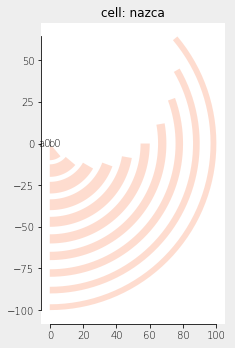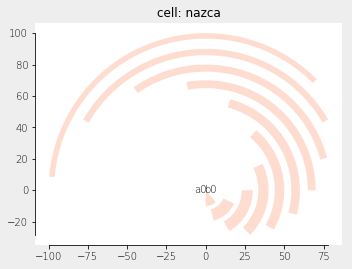Home Forums Nazca Questions and Answers Concentric circles

Tagged: ,

Viewing 2 posts - 1 through 2 (of 2 total)
• Author
Posts
• #5672

Hello Nazca Team,

I want to draw concentric circles with certain line thickness, constant turning space and gradually increasing/decreasing turning space as in this figure (middle one). I couldn’t figure out how to do it and would be glad if you can help me.

Gozde

#5674

Dear Gozde,

You can use Nazca Polygon objects for that, which you feed with “normal” polygons [(x1, y1), (x2, y2), … ]. There is a helper module nazca.geometries with methods which generate polygons for various shapes to make life easier.

``````import nazca as nd
import nazca.geometries as geom

for i in range(10):
points = geom.arc(radius=10*i, width=8-0.5*i, angle=40+10*i, N=50)
nd.Polygon(points=points, layer=1).put(0)

nd.export_plt()``````With some extra rotation:

``````for i in range(10):
points = geom.arc(radius=10+10*i, width=8-0.5*i, angle=40+10*i, N=50)
nd.Polygon(points=points, layer=1).put(0, 0, i*15)

nd.export_plt()``````Ronald

Viewing 2 posts - 1 through 2 (of 2 total)
• You must be logged in to reply to this topic.